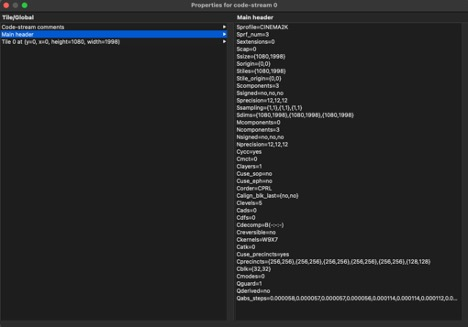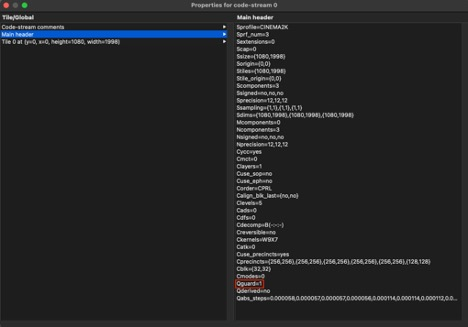# RDD52 Guardbit requirements of JPEG 2000

Jack Watts | May 18, 2023 min read

What is a guard bit? And how do I know what is set in my encoded JPEG 200 image.

There are two freely available tools that will produce you the Guard Bit Information of a JPEG 2000 image.

OpenJPEG
Distributed under the open source project OpenJPEG. The included CLI utili opj_dump will produce a STDOUT dump.
Kdu_winshow or kdu_macshow is a lightweight UI based JPEG 200 inspector tool.

## Background

Understanding the SMPTE RDD 52 constraints on ISO 15444-1 2016.

NOTE: Remember that the 2016 standdard is explicitly referneced for D-Cinema applications.

The number of guard bits to be specified in the QCD marker shall be a value of 1 for 2K content, and shall be a value of 2 for 4K content.

QCD: Quantization Default describes the quantization default used for compressing all components. It is located in the main and first tile-part header of a given tile. This is applied to all components, each component being a tile-part of a given single tile, thus aligning with the RDD 52 constraint “the entire image shall be encoded as a single tile.” As such, there is a guard bit value for each component. This is summarized and presented differently in each of the three aforementioned tools which I will now detail.

1. Open you j2c image in kdu_show
2. Then go to `Main Menu > File > Properties`1. In the Properties dialog, select `Main Header`. The right hand side of the UI will now populate with similar info pertaining to the j2c file.Here `kdu_show` displays it differently, as all components = a part-tile of a main tile it displays the guard bit info (Qguard) for the main tile. Here it is set to ‘1’ which is correct for a 2K image.

Note: This would be set to ‘2’ if 4K.

## OpenJPEG

Run the following command

`opj_dump -i <path-to-j2c.j2k>`

``````[INFO] Start to read j2k main header (0).
[INFO] Main header has been correctly decoded.
Image info {
x0=0, y0=0
x1=1998, y1=1080
numcomps=3
component 0 {
dx=1, dy=1
prec=12
sgnd=0
}
component 1 {
dx=1, dy=1
prec=12
sgnd=0
}
component 2 {
dx=1, dy=1
prec=12
sgnd=0
}
}
Codestream info from main header: {
tx0=0, ty0=0
tdx=1998, tdy=1080
tw=1, th=1
default tile {
csty=0x1
prg=0x4
numlayers=1
mct=1
comp 0 {
csty=0x1
numresolutions=6
cblkw=2^5
cblkh=2^5
cblksty=0
qmfbid=0
preccintsize (w,h)=(7,7) (8,8) (8,8) (8,8) (8,8) (8,8)
qntsty=2
numgbits=1
stepsizes (m,e)=(1844,15) (1777,15) (1777,15) (1710,15) (1777,14) (1777,14) (1710,14) (1794,13) (1794,13) (1760,13) (1869,12) (1869,12) (1895,12) (3,10) (3,10) (68,10)
roishift=0
}
comp 1 {
csty=0x1
numresolutions=6
cblkw=2^5
cblkh=2^5
cblksty=0
qmfbid=0
preccintsize (w,h)=(7,7) (8,8) (8,8) (8,8) (8,8) (8,8)
qntsty=2
numgbits=1
stepsizes (m,e)=(1844,15) (1777,15) (1777,15) (1710,15) (1777,14) (1777,14) (1710,14) (1794,13) (1794,13) (1760,13) (1869,12) (1869,12) (1895,12) (3,10) (3,10) (68,10)
roishift=0
}
comp 2 {
csty=0x1
numresolutions=6
cblkw=2^5
cblkh=2^5
cblksty=0
qmfbid=0
preccintsize (w,h)=(7,7) (8,8) (8,8) (8,8) (8,8) (8,8)
qntsty=2
numgbits=1
stepsizes (m,e)=(1844,15) (1777,15) (1777,15) (1710,15) (1777,14) (1777,14) (1710,14) (1794,13) (1794,13) (1760,13) (1869,12) (1869,12) (1895,12) (3,10) (3,10) (68,10)
roishift=0
}
}
}
Codestream index from main header: {
Marker list: {
type=0xff4f, pos=0, len=2
type=0xff51, pos=2, len=49
type=0xff52, pos=51, len=20
type=0xff5c, pos=71, len=37
type=0xff55, pos=108, len=21
type=0xff64, pos=129, len=42
}
}
``````

As before, `opj_dump` displays the same info differently, this time opting for a component by component layout. As you can see in the highlighted output, per component, “numgbits” is set to ‘1’.

Note: This would be set to 2 if 4K.